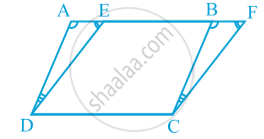Share

# Parallelograms on the Same Base and Between the Same Parallels

#### theorem

Theorem: Parallelograms on the same base and between the same parallels are equal in area.
Proof : Two parallelograms ABCD and EFCD, on the same base DC and between the same parallels AF and DC are given in following fig.We need to prove that ar (ABCD) = ar (EFCD).
In ∆ ADE and ∆ BCF,
∠ DAE = ∠ CBF (Corresponding angles from AD || BC and transversal AF)   (1)
∠ AED = ∠ BFC (Corresponding angles from ED || FC and transversal AF) (2)
Therefore, ∠ ADE = ∠ BCF (Angle sum property of a triangle) (3)
Also, AD = BC (Opposite sides of the parallelogram ABCD) (4)
So, ∆ ADE ≅ ∆ BCF         [By ASA rule, using (1), (3), and (4)]
Therefore, ar (ADE) = ar (BCF) (Congruent figures have equal areas) (5)
Now, ar (ABCD) = ar (ADE) + ar (EDCB)
= ar (BCF) + ar (EDCB)         [From(5)]
= ar (EFCD)
So, parallelograms ABCD and EFCD are equal in area.

### Shaalaa.com

Theorem : Parallelograms on the same base and between the same parallels are equal in area. [00:08:31]
S
0%

S